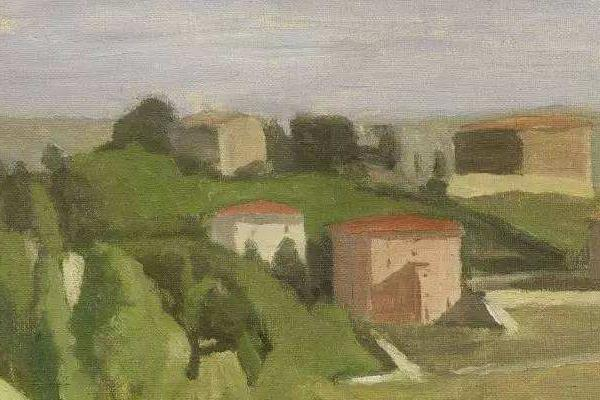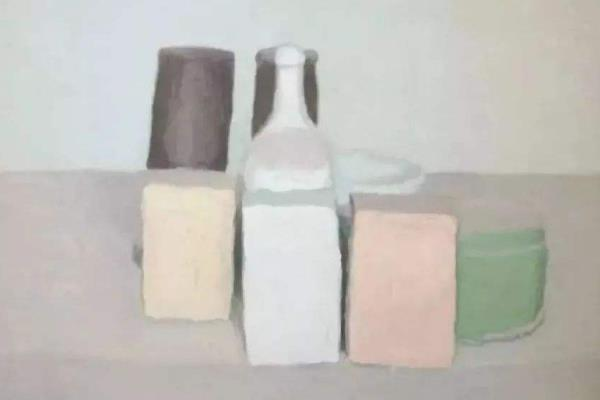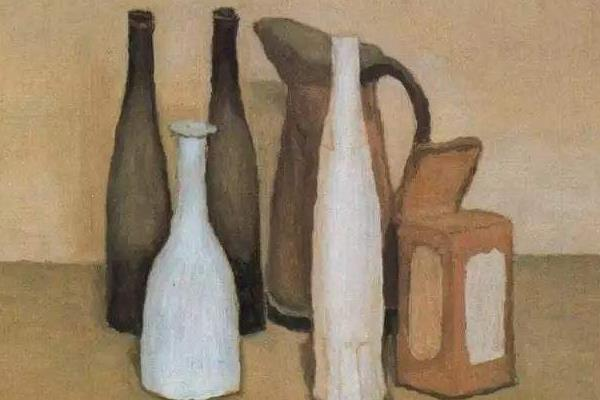2018-12-02

# 高级灰是一种色调介于明亮与暗淡之间的中间色，它的特点是加在任何中间层次的颜色中都不会使总体色调显得过亮或过暗。水粉画和油画中长运用高级灰色来表现画面，高级灰的调和需要绘画者在长期的实践练习中总结经验。在油画中常出现高级灰的色调，高级灰只能隐隐约约看得出总体色相，有一种灰亮感。高级灰的调法需要一定的油画基础与技巧，加上反复的练习才能做到。常用的高级灰的调色方法有：果子黄=中黄+肉色、黄灰=肉色+柠檬黄+白+天蓝（一点）、埃及灰=土黄+橄榄绿+白、天鹅灰=灰+粉绿+天蓝+白、冷固灰=土黄+黄绿、高光灰=白＋天蓝、蓝灰=粉绿+湖蓝＋白、冰梨灰=橄榄绿+中黄+土黄、紫灰=玫瑰红+天蓝+白、苹果灰=黄绿+中黄、水晶灰=橄榄绿+白、绿灰=橄+黄绿+白、绿油灰=墨绿+土黄+玫、暖绿=中绿（多）+深红（少）、香蕉灰=柠+黄绿+白、土陶灰=赭石+紫罗兰+墨绿暖梨灰=土黄+橄榄绿、太平洋灰=青莲+天蓝+普兰+粉绿、瓦蓝会=普蓝+粉绿+紫罗兰（少)灰色理想兰=湖蓝+玫瑰、西瓜红=大红+柠檬黄、灰色理想紫=玫瑰红+天蓝、冷重灰=黑(稀释）+普兰、暖重灰=黑(稀释）+深红、那坡里黄=白（多）+柠檬黄+肉色、祜蓝灰=祜蓝+粉绿+天蓝土儿黄=赭石+肉色+玫瑰（一点)、红果灰=肉色（多）+橘红+深红+天蓝、成灰=肉色+橘红、红油灰=深红+橄榄绿等等。## 调配高级灰时的注意事项